Courses

# Principle of Superposition - 2 Civil Engineering (CE) Notes | EduRev

## Civil Engineering (CE) : Principle of Superposition - 2 Civil Engineering (CE) Notes | EduRev

The document Principle of Superposition - 2 Civil Engineering (CE) Notes | EduRev is a part of the Civil Engineering (CE) Course Structural Analysis.
All you need of Civil Engineering (CE) at this link: Civil Engineering (CE)

Consider a member of constant cross sectional area A , subjected to axial force P as shown in Fig. 2.8. Let E be the Young’s modulus of the material. Let the member be under equilibrium under the action of this force, which is applied through the centroid of the cross section. Now, the applied force P is resisted by uniformly distributed internal stresses given by average stress σ = P/A as shown by the free body diagram (vide Fig. 2.8). Under the action of axial load P applied at one end gradually, the beam gets elongated by (say) u. This may be calculated as follows. The incremental elongation du of small element of length of beam is given by,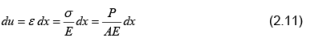Now the total elongation of the member of length L may be obtained by integration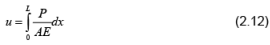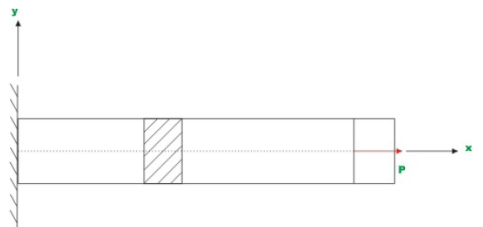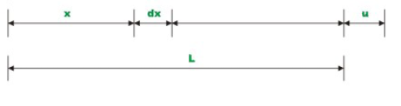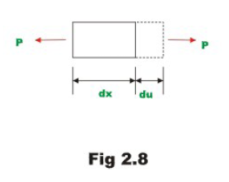Now the work done by external loads w = 1/2 Pu                                                           (2.13)

In a conservative system, the external work is stored as the internal strain energy. Hence, the strain energy stored in the bar in axial deformation is,

U =1/2 Pu                                                     (2.14)

Substituting equation (2.12) in (2.14) we get,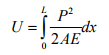(2.15)

Strain energy due to bending

Consider a prismatic beam subjected to loads as shown in the Fig. 2.9. The loads are assumed to act on the beam in a plane containing the axis of symmetry of the cross section and the beam axis. It is assumed that the transverse cross sections (such as AB and CD), which are perpendicular to centroidal axis, remain plane and perpendicular to the centroidal axis of beam (as shown in Fig 2.9).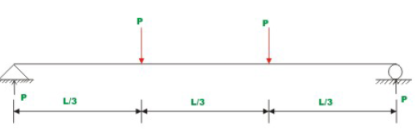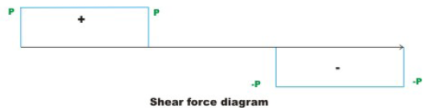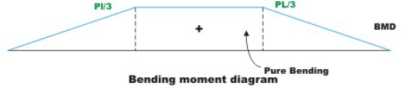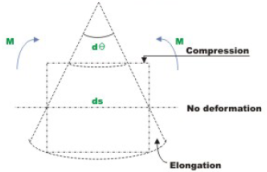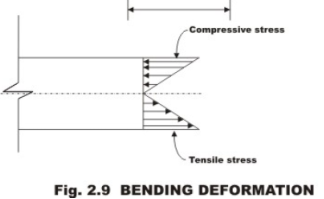Consider a small segment of beam of length ds subjected to bending moment as shown in the Fig. 2.9. Now one cross section rotates about another cross section by a small amount ds dθ. From the figure,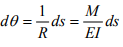(2.16)

where R is the radius of curvature of the bent beam and EI is the flexural rigidity of the beam. Now the work done by the moment M while rotating through angle dθ will be stored in the segment of beam as strain energy dU. Hence,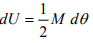(2.17)

Substituting for dθ in equation (2.17), we get,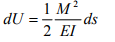(2.18)

Now, the energy stored in the complete beam of span L may be obtained by integrating equation (2.18). Thus,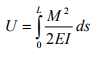(2.19)

Strain energy due to transverse shear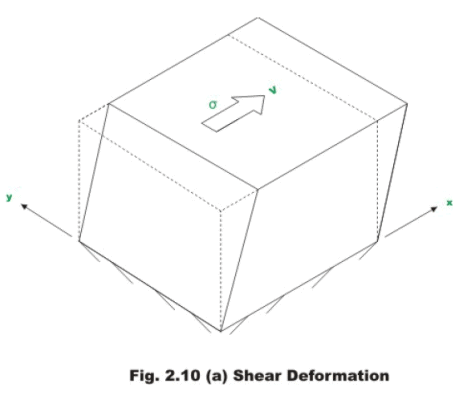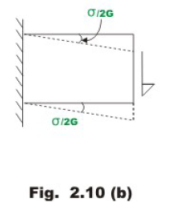The shearing stress on a cross section of beam of rectangular cross section may be found out by the relation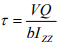(2.20)

where Q is the first moment of the portion of the cross-sectional area above the point where shear stress is required about neutral axis, V is the transverse shear force, b is the width of the rectangular cross-section and Izz is the moment of inertia of the cross-sectional area about the neutral axis. Due to shear stress, the angle between the lines which are originally at right angle will change. The shear stress varies across the height in a parabolic manner in the case of a rectangular cross-section. Also, the shear stress distribution is different for different shape of the cross section. However, to simplify the computation shear stress is assumed to be uniform (which is strictly not correct) across the cross section. Consider a segment of length ds subjected to shear stress τ . The shear stress across the cross section may be taken as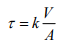in which A is area of the cross-section and k is the form factor which is dependent on the shape of the cross section. One could write, the deformation du as

du = Δ γ ds                                         (2.21)

where Δγ is the shear strain and is given by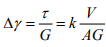(2.22)

Hence, the total deformation of the beam due to the action of shear force is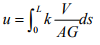(2.23)

Now the strain energy stored in the beam due to the action of transverse shear force is given by,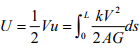(2.24)

The strain energy due to transverse shear stress is very low compared to strain energy due to bending and hence is usually neglected. Thus the error induced in assuming a uniform shear stress across the cross section is very small.

Strain energy due to torsion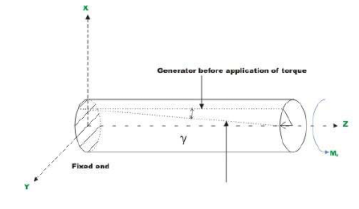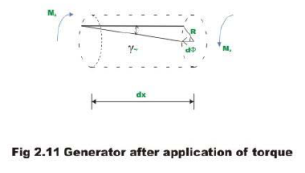Consider a circular shaft of length L radius R , subjected to a torque T at one end (see Fig. 2.11). Under the action of torque one end of the shaft rotates with respect to the fixed end by an angle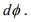Hence the strain energy stored in the shaft is,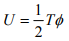(2.25)

Consider an elemental length ds of the shaft. Let the one end rotates by a small amount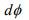with respect to another end. Now the strain energy stored in the elemental length is,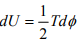(2.26)

We know that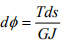(2.27)

where, G is the shear modulus of the shaft material and J is the polar moment of area. Substituting forfrom (2.27) in equation (2.26), we obtain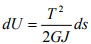(2.28)

Now, the total strain energy stored in the beam may be obtained by integrating the above equation.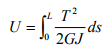(2.29)

Hence the elastic strain energy stored in a member of length s (it may be curved or straight) due to axial force, bending moment, shear force and torsion is summarized below.

1. Due to axial force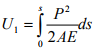2. Due to bending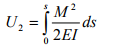3. Due to shear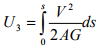4. Due to torsion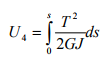Summary

In this lesson, the principle of superposition has been stated and proved. Also, its limitations have been discussed. In section 2.3, it has been shown that the elastic strain energy stored in a structure is equal to the work done by applied loads in deforming the structure. The strain energy expression is also expressed for a 3- dimensional homogeneous and isotropic material in terms of internal stresses and strains in a body. In this lesson, the difference between elastic and inelastic strain energy is explained. Complementary strain energy is discussed. In the end, expressions are derived for calculating strain stored in a simple beam due to axial load, bending moment, transverse shear force and torsion.

Offer running on EduRev: Apply code STAYHOME200 to get INR 200 off on our premium plan EduRev Infinity!

## Structural Analysis

30 videos|122 docs|28 tests

,

,

,

,

,

,

,

,

,

,

,

,

,

,

,

,

,

,

,

,

,

;